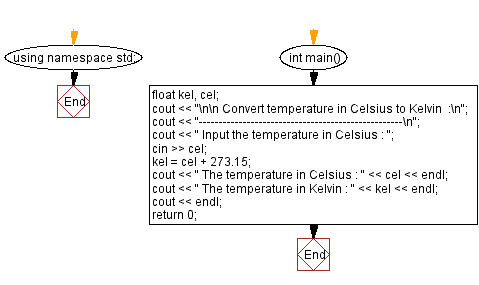﻿ C++ Exercises: Convert temperature in Celsius to Kelvin - w3resource

# C++ Exercises: Convert temperature in Celsius to Kelvin

## C++ Basic: Exercise-27 with Solution

Write a program in C++ to convert temperature in Celsius to Kelvin.

Sample Solution:

C++ Code :

``````#include <iostream>
using namespace std;

int main()
{
float kel, cel;
cout << "\n\n Convert temperature in Celsius to Kelvin  :\n";
cout << "---------------------------------------------------\n";
cout << " Input the temperature in Celsius : ";
cin >> cel;
kel = cel + 273.15;
cout << " The temperature in Celsius : " << cel << endl;
cout << " The temperature in Kelvin : " << kel << endl;
cout << endl;
return 0;
}
``````

Sample Output:

``` Convert temperature in Celsius to Kelvin  :
---------------------------------------------------
Input the temperature in Celsius : 26.85
The temperature in Celsius : 26.85
The temperature in Kelvin : 300
```

Flowchart:C++ Code Editor:

What is the difficulty level of this exercise?

﻿

## C++ Programming: Tips of the Day

Why is there no std::stou?

The most pat answer would be that the C library has no corresponding "strtou", and the C++11 string functions are all just thinly veiled wrappers around the C library functions: The std::sto* functions mirror strto*, and the std::to_string functions use sprintf.

Ref: https://bit.ly/3wtz2qA

We are closing our Disqus commenting system for some maintenanace issues. You may write to us at reach[at]yahoo[dot]com or visit us at Facebook